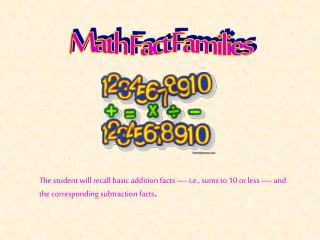Download PresentationMath Fact Families

# Math Fact Families - PowerPoint PPT Presentation

Math Fact Families. The student will recall basic addition facts — i.e., sums to 10 or less — and the corresponding subtraction facts. Checking What You Know Are the following math sentences the same?. 3 + 2 =. 5. 2 + 3 =. 5. 4 + 6 =. 10. 6 + 4 =. 10. 1 + 7 =. 8. 7 + 1 =. 8. +1.I am the owner, or an agent authorized to act on behalf of the owner, of the copyrighted work described.
Download Presentation## Math Fact Families

Download Policy: Content on the Website is provided to you AS IS for your information and personal use and may not be sold / licensed / shared on other websites without getting consent from its author.While downloading, if for some reason you are not able to download a presentation, the publisher may have deleted the file from their server.

- - - - - - - - - - - - - - - - - - - - - - - - - - E N D - - - - - - - - - - - - - - - - - - - - - - - - - -
Presentation Transcript
1. Math Fact Families The student will recall basic addition facts — i.e., sums to 10 or less — and the corresponding subtraction facts.

2. Checking What You Know Are the following math sentences the same? 3 + 2 = 5 2 + 3 = 5

3. 4 + 6 = 10 6 + 4 = 10

4. 1 + 7 = 8 7 + 1 = 8

5. +1

6. 1 + 1 = 2 1 2 - 1 =

7. 2 + 1 = 3 2 3 - 1 = 1 3 - 2 =

8. 3 + 1 = 4 4 - 1 = 3 4 - 3 = 1

9. 4 + 1 = 5 4 5 - 1 = 5 - 4 = 1

10. 5 + 1 = 6 5 6 - 1 = 1 6 - 5 =

11. 7 + 1 = 8 7 8 - 1 = 1 8 - 7 =

12. 8 + 1 = 9 8 9 - 1 = 1 9 - 8 =

13. 9 + 1 = 10 9 10 - 1 = 10 - 9 = 1

14. +2

15. 1 + 2 = 3 3 - 1 = 2 3 - 2 = 1

16. 2 + 2 = 4 2 4 - 2 =

17. 3 + 2 = 5 5 - 2 = 3 5 - 3 = 2

18. 4 + 2 = 6 2 6 - 4 = 6 - 2 = 4

19. 5 + 2 = 7 5 7 - 2 = 2 7 - 5 =

20. 6 + 2 = 8 6 8 - 2 = 2 8 - 6 =

21. 7 + 2 = 9 7 9 - 2 = 2 9 - 7 =

22. 8 + 2 = 10 8 10 - 2 = 2 10 - 8 =

23. +3

24. 1 + 3 = 4 4 - 1 = 3 4 - 3 = 1

25. 2 + 3 = 5 3 5 - 2 = 2 5 - 3 =

26. 3 + 3 = 6 6 - 3 = 3

27. 4 + 3 = 7 3 7 - 4 = 7 - 3 = 4

28. 5 + 3 = 8 5 8 - 3 = 3 8 - 5 =

29. 6 + 3 = 9 6 9 - 3 = 3 9 - 6 =

30. 7 + 3 = 10 7 10 - 3 = 3 10 - 7 =

31. +4

32. 1 + 4 = 5 5 - 1 = 4 5 - 4 = 1

33. 2 + 4 = 6 4 6 - 2 = 2 6 - 4 =

34. 3 + 4 = 7 4 7 - 3 = 7 - 3 = 4

35. 4 + 4 = 8 4 8 - 4 =

36. 5 + 4 = 9 5 9 - 4 = 4 9 - 5 =

37. 6 + 4 = 10 6 10 - 4 = 4 10 - 6 =

38. +5

39. 1 + 5 = 6 6 - 1 = 5 6 - 5 = 1

40. 2 + 5 = 7 5 7 - 2 = 2 7 - 5 =

41. 3 + 5 = 8 5 8 - 3 = 8 - 5 = 3

42. 4 + 5 = 9 5 9 - 4 = 4 9 - 5 =

43. 5 + 5 = 10 5 10 - 5 =

44. +6

45. 1 + 6 = 7 7 - 1 = 6 7 - 6 = 1

46. 2 + 6 = 8 6 8 - 2 = 2 8 - 6 =

47. 3 + 6 = 9 6 9 - 3 = 9 - 6 = 3

48. 4 + 6 = 10 6 10 - 4 = 4 10 - 6 =

49. +7

50. 1 + 7 = 8 8 - 1 = 7 8 - 7 = 1